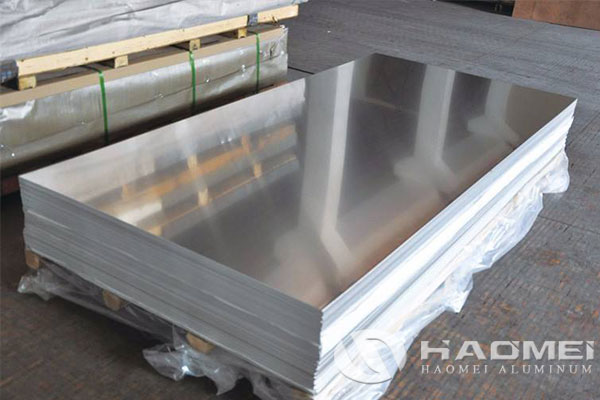Welcome to the web of Haomei Aluminum

# 2mm Aluminium Sheet Weight

### January 18, 20232mm aluminium sheet weightweight of 2mm aluminium sheet

Calculate 2mm aluminium sheet weight with formula provide by aluminum sheet manufacturer with be easier. The weight of aluminium sheet can be calculated by the volume of the aluminum sheet multiplied by the density of aluminum – 2700kg per cubic meter. Weight of aluminium sheet =aluminum sheet area × aluminium sheet thickness × specific gravity, if the unit of weight is kilogram, the unit of length should be converted into decimeter. For example: 1 square meter aluminum sheet is 100 square decimeters, 3 mm thickness is 0.03 decimeters, and the specific gravity of aluminum is 2.7 kg/cubic decimeters. Weight = 100 × 0.03 × 2.7 = 8.1 kg, namely: 3 mm thick aluminum sheet weighs 8.1 kg per square meter.For 2mm aluminium sheet weight, the weight of a square meter is 5400 grams, or 5.4 kg. A square meter of 2 millimeter of aluminium sheet means an aluminum sheet with a bottom area of 1 square meter (ie 10,000 square centimeters) and a thickness (height) of 2 millimeter (ie 0.2 cm). The volume of this aluminium sheet is: V=Sh=10000cm2x0.2cm=2000cm3. The density of aluminum is 2.7 g/cm3. 2.7 grams per cubic centimeter x 2000 cubic centimeters = 5400 grams, or 5.4 kilograms (kg).

After calculate 2mm aluminium sheet weight, let take 1mm aluminum sheet weight as other example, 0ne millimeter of aluminum sheet weighs 2700 grams per square, or 2.7 kilograms. A square of one millimeter of aluminum sheet means an aluminum sheet with a bottom area of 1 square meter (ie 10,000 square centimeters) and a thickness (height) of 1 millimeter (ie 0.1 cm). The volume of this aluminum sheet is: V=Sh=10000cm2x0.1cm=1000cm3. The density of aluminum is 2.7 g/cm3. 2.7 grams per cubic centimeter x 1000 cubic centimeters = 2700 grams, or 2.7 kilograms (kg).